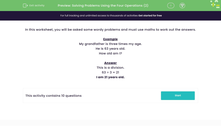# Solve Word Problems Using the Four Operations

In this worksheet, students will use their knowledge of addition, subtraction, multiplication and division to solve a range of problems.Key stage:  KS 2

Curriculum topic:   Number: Multiplication and Division

Curriculum subtopic:   Solve Multiplication/Division Problems

Difficulty level:#### Worksheet Overview

In this activity, we will work on word problems using addition, subtraction, multiplication, and division.

Before starting to solve a word problem, we must check which operation is needed.

Let's take a look at a word problem together.

Sam has 12 stickers.

James has three times as many as Jake.

How many does James have?

This question is a multiplication word problem.

We need to triple the number of stickers Jake has, to find out how many Jane has.

To triple a number, we multiply it by 3.

12 × 3 = 36

James has 36 stickers.Now let's try an example question together.

Example

My grandfather is three times my age.

He is 63 years old.

How old am I?

This is a division problem.

63 ÷ 3 = 21

I am 21 years old.Now you're ready to try some questions by yourself.

### What is EdPlace?

We're your National Curriculum aligned online education content provider helping each child succeed in English, maths and science from year 1 to GCSE. With an EdPlace account you’ll be able to track and measure progress, helping each child achieve their best. We build confidence and attainment by personalising each child’s learning at a level that suits them.

Get started